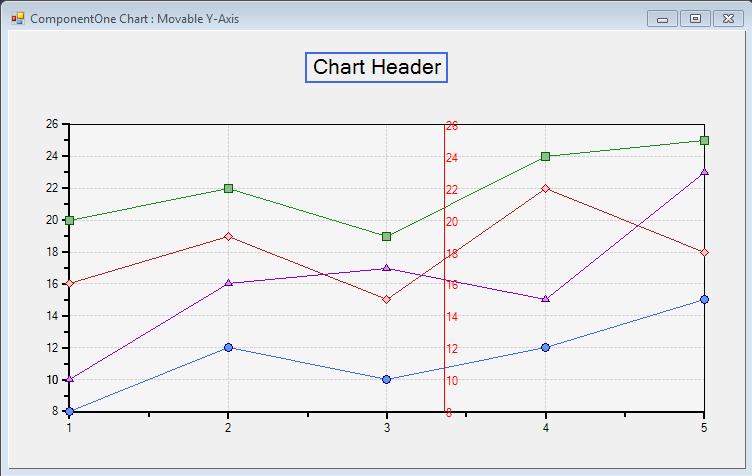Our Winforms Charts provide support for tooltips. In this blog we will discuss way to display movable Y-Axis as chart tooltip. The implementation is quite simple. We need to capture the data points and then create a false Y-Axis over the chart. To start with first we need to capture the position of the mouse when it hovers over the chart. We will save the X and Y position using Move event of C1Chart,

``````private void c1Chart1_MouseMove(object sender, MouseEventArgs e)
{
X = e.X;
Y = e.X;
Invalidate(true);
}
``````

Next thing to do is to create a false Y-Axis which can be displayed over the chart. For this we will handle the Paint event of C1Chart and draw a line using Graphics.DrawLine() method.

``````Graphics g = e.Graphics;
g.DrawLine(pen, X, c1Chart1.ChartArea.PlotArea.Location.Y, X, c1Chart1.ChartArea.PlotArea.Location.Y + c1Chart1.ChartArea.PlotArea.Size.Height);
``````

Next we need to populate this line with the Chartdataseries points for the Y-Axis and draw the values over the false Y-Axis. So our Paint event would be as follows :

``````private void c1Chart1_Paint(object sender, PaintEventArgs e)
{
if (X > 75 && X < 700)
{
Graphics g = e.Graphics;
g.DrawLine(pen, X, c1Chart1.ChartArea.PlotArea.Location.Y, X, c1Chart1.ChartArea.PlotArea.Location.Y + c1Chart1.ChartArea.PlotArea.Size.Height);
double numofpoints = c1Chart1.ChartArea.AxisY.Max - c1Chart1.ChartArea.AxisY.Min;
double unitmajor = c1Chart1.ChartArea.AxisY.UnitMajor;
double ymin = c1Chart1.ChartArea.AxisY.Min;

float x = 0, ypoint = 0;

for (int i = 0; i <= (int)numofpoints / unitmajor; i++)
{
c1Chart1.ChartGroups.DataCoordToCoord(0, ymin + (i * unitmajor), ref x, ref ypoint);
g.DrawString((ymin + (i * unitmajor)).ToString(), new Font("Microsoft Sans Serif", 8.25f), new SolidBrush(Color.Red), X, ypoint - 5);
ypoint = ypoint - (25 * (float)unitmajor);
}

}
}
``````

Now when we on hovering mouse over C1Chart, we can see a movable Y-Axis in the tooltip.Download Sample